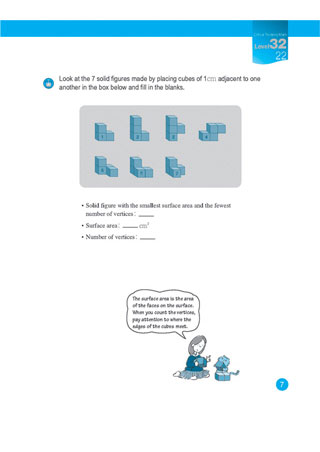## EYE LEVEL MATH

Eye Level Math will take your child through a study area covering the basics of numbers
all the way through critical thinking math such as algebra and statistics.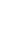Play Math %>

Watch the video(s) above to find out what Eye Level Math is all about.

### CONCEPT & BENEFITS

• Foundation in
5 fundamental areas
of math
• Master each math concept through a small step approach
• Develop critical and analytical thinking skills.
Offline Learning

Learn new concept on paper first

Practice solving problems with guidance

Use supplemental materials to improve problem solving skills

Check progress with interim assessments

Online Learning

Practice newly learned concepts with additional problems

Receive instant feedback through an automated scoring system

Play fun interactive games

Track study records

Be exposed to the benefits of blended learning

MASTERY IN MATH

### CURRICULUM DESIGN

BASIC THINKING MATH

Building basic foundation of arithmetic skills

LEARNING METHOD
Online & Offline
Numbers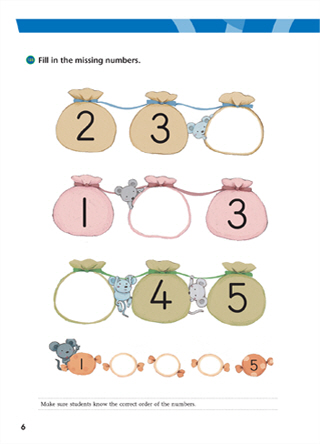Arithmetic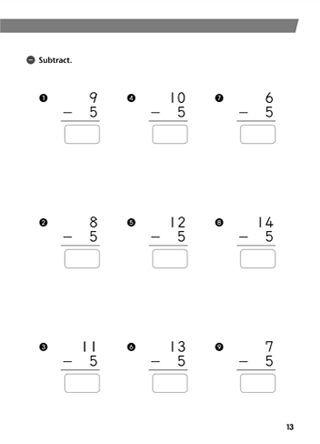Measurement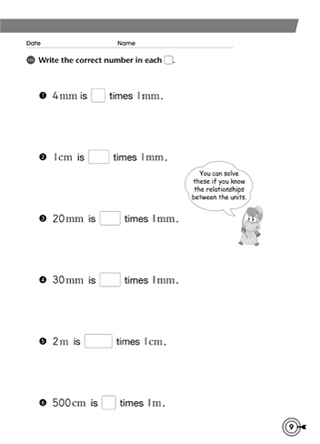Equations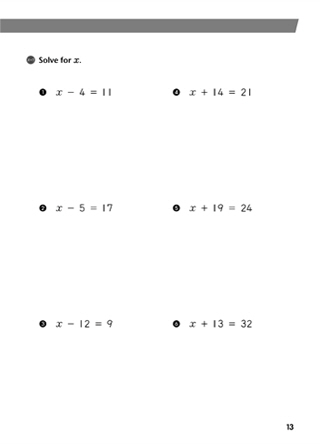Numbers and Arithmetic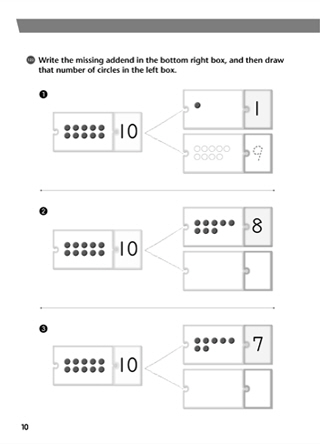Variables and Equations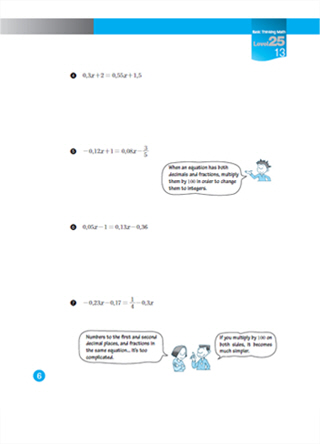Probability and Statistics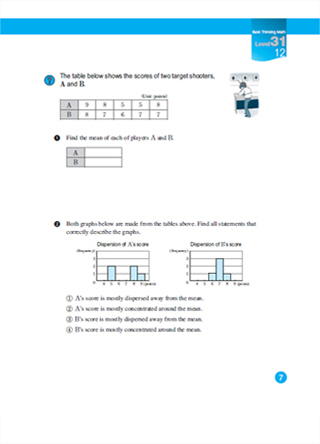Geometry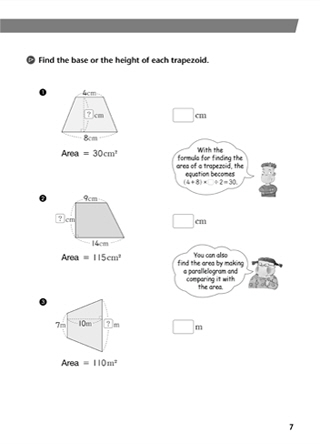CRITICAL THINKING MATH

Developing problem solving, patterns, geometry, measurement and reasoning skills in advanced mathematical skills.

LEARNING METHOD
Offline
Relationships and Functions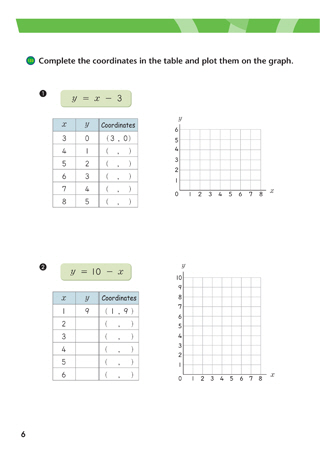Patterns and Relationships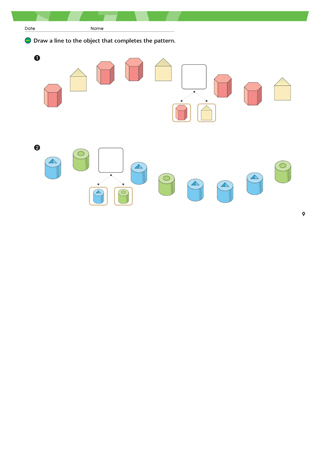Measurement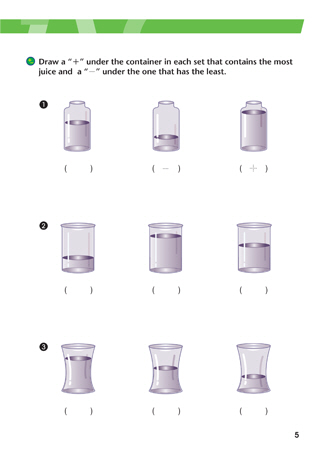Geometry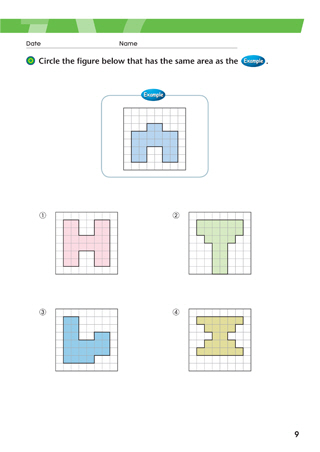Reasoning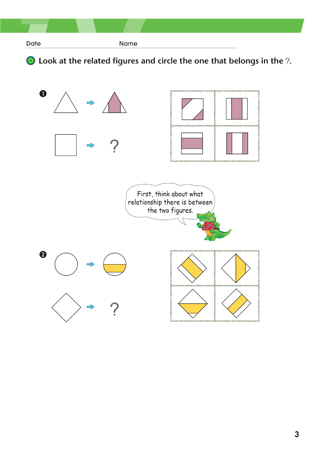Problem Solving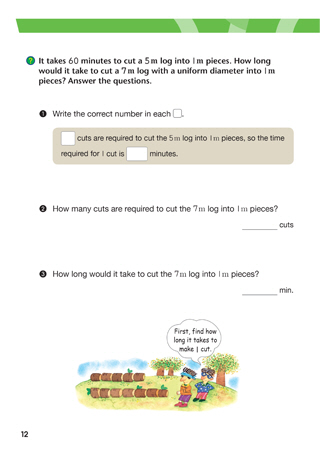Special Sense